# Texas Go Math Grade 2 Lesson 19.6 Answer Key Graphs: Scales of 2 or More

Refer to our Texas Go Math Grade 2 Answer Key Pdf to score good marks in the exams. Test yourself by practicing the problems from Texas Go Math Grade 2 Lesson 19.6 Answer Key Graphs: Scales of 2 or More.

## Texas Go Math Grade 2 Lesson 19.6 Answer Key Graphs: Scales of 2 or More

Essential Question
How can you make a graph that uses a scale of 2 or more?

1. Choose an appropriate scale for the x- and y-axis.
2. Plot the points accurately.
3. Label the both axes including a brief description of the data and the units.
4. Give your graph a suitable title.
5. State the source of data

Explore
Use the graph to solve.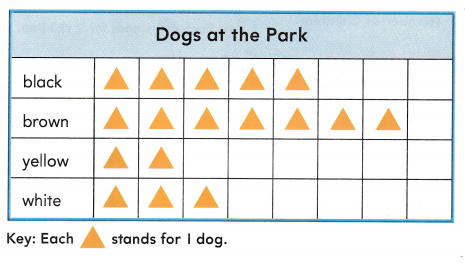___ brown dogs
Explanation:
There are 7 brown dogs

For The Teacher

• Read the following problem. Jason and his dad went to the park. They saw these dogs at the park. How many brown dogs did they see at the park?
They saw 7 Brown dogs in the park.

Math Talk
Mathematical Processes
How many brown dogs and white dogs are at the park? Explain.
Explanation:
There are 7 Brown dogs and 3 white dogs
so, there are 10 dogs and white dogs are at the park.

Model and Draw
Use the data in the bar graph to make a pictograph. Look closely at the scale and key of the graphs.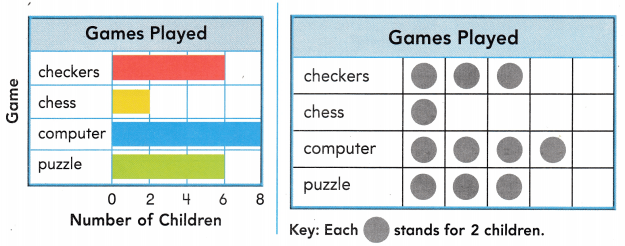Explanation:
The pictograph is done

Share and Show

Question 1.
Use the data in the pictograph to make a bar graph.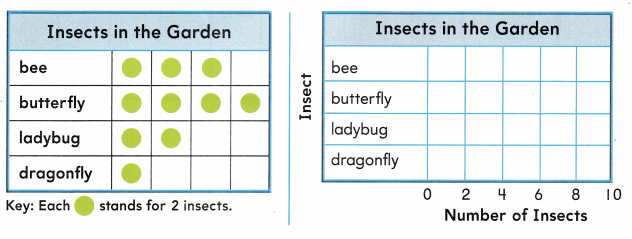Question 2.
Explanation:
There are 4 ladybugs in the garden

Question 3.
How many more bees than ladybugs are in the garden? ____ more bees
bees = 6
6 – 4 = 2
Explanation:
There are 2 more bees than ladybugs

Problem Solving

Question 4.
H.O.T. Multi-Step Use the data in the bar graph to make a pictograph.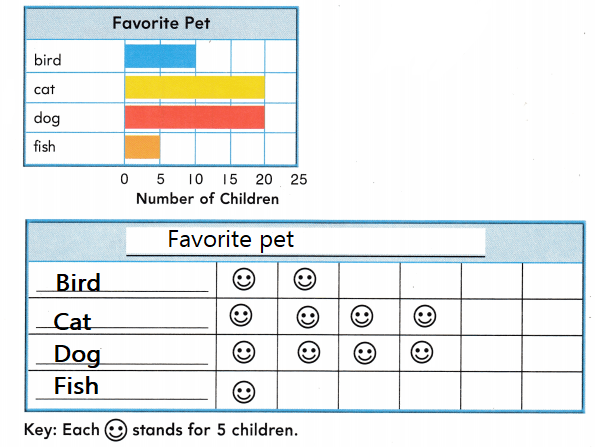Question 5.
Look at the graphs above. Ted thinks that 2 more children chose dog than bird. Is Ted correct? Explain.
Explanation:
By the graph we can see that
The children chose bird is 10
and the children who chose dog are 20
so, Dogs 10 more than the bird.

Question 6.
Use the key. Draw pictures in the pictograph to show the data.
There are 10 white cats Cots at the cat show.

There are 6 black cats at the cat show.

There are 4 brown cats at the cat show.Question 7.
Representations Use the pictograph. How many fewer brown cats than black cats are at the cat show?
(A) 4
(B) 1
(C) 2
Number of brown cats = 4
Number of black cats = 6
6 – 4 = 2Question 8.
Texas Test Prep Use the bar graph. How many books did Noah read?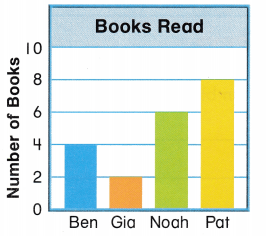(A) 3
(B) 6
(C) 4
Explanation:

### Texas Go Math Grade 2 Lesson 19.6 Homework and Practice Answer Key

Question 1.
Use the data in the pictograph to make a bar graph.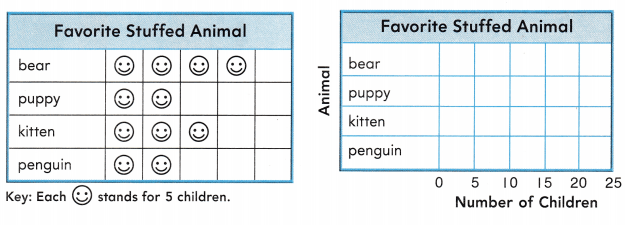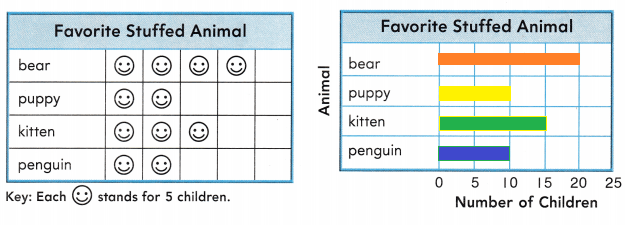Explanation:
With the help of pictograph bar graph was done.

Question 2.
How many children chose puppy or bear?
___ children
Explanation:
30 children chose puppy or bear
puppy = 10
Bear = 20
20 + 10 = 30

Problem Solving

Use the graphs above.

Question 3.
Multi-Step Andy thinks that I fewer child chose kitten than bear. Is Andy correct? Explain.
Explanation:
Andy thinks that I fewer child chose kitten than bear.
but Number of kittens are 15
and number of bears are 20
20 – 15 = 5
so , 5 fewer bears

Texas Test Prep

Lesson Check

Question 4.
Uše the bar graph. How many flag stickers does Kyle have?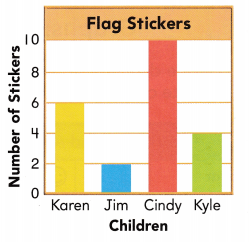(A) 3
(B) 6
(C) 4
Explanation:
Kyle have 4 fewer stickers

Rosa makes a pictograph to record the color of bikes she sees at the park.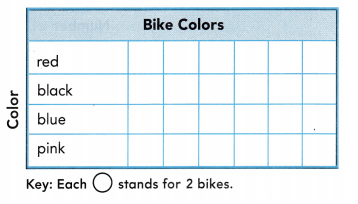Question 5.
There are 8 black bikes at the park. How manywill Rosa draw to show the black bikes?824
Explanation:
She draw 4 because 4 x 2 = 8= 2

Question 6.
Rosa draws 3in the row for pink bikes. How many pink bikes does she see at the park?
(A) 6
(B) 2
(C) 3= 2